# Test: Noise - Electronics and Communication Engineering (ECE)

Test Description

## 20 Questions MCQ Test GATE ECE (Electronics) 2024 Mock Test Series - Test: Noise

Test: Noise for Electronics and Communication Engineering (ECE) 2023 is part of GATE ECE (Electronics) 2024 Mock Test Series preparation. The Test: Noise questions and answers have been prepared according to the Electronics and Communication Engineering (ECE) exam syllabus.The Test: Noise MCQs are made for Electronics and Communication Engineering (ECE) 2023 Exam. Find important definitions, questions, notes, meanings, examples, exercises, MCQs and online tests for Test: Noise below.
Solutions of Test: Noise questions in English are available as part of our GATE ECE (Electronics) 2024 Mock Test Series for Electronics and Communication Engineering (ECE) & Test: Noise solutions in Hindi for GATE ECE (Electronics) 2024 Mock Test Series course. Download more important topics, notes, lectures and mock test series for Electronics and Communication Engineering (ECE) Exam by signing up for free. Attempt Test: Noise | 20 questions in 60 minutes | Mock test for Electronics and Communication Engineering (ECE) preparation | Free important questions MCQ to study GATE ECE (Electronics) 2024 Mock Test Series for Electronics and Communication Engineering (ECE) Exam | Download free PDF with solutions
 1 Crore+ students have signed up on EduRev. Have you?
Test: Noise - Question 1

### An amplifier has a standard spot noise figure F0  = 6.31 (8.0 dB). The amplifier, that is used to amplify the output of an antenna have antenna temperature of Ta = 180 K Que: The effective input noise temperature of this amplifier is

Detailed Solution for Test: Noise - Question 1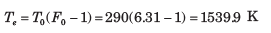Test: Noise - Question 2

### An amplifier has a standard spot noise figure F0  = 6.31 (8.0 dB). The amplifier, that is used to amplify the output of an antenna have antenna temperature of Ta = 180 K Que: The operating spot noise figure is

Detailed Solution for Test: Noise - Question 2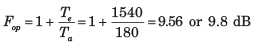Test: Noise - Question 3

### An amplifier has three stages for which Te1 = 200 K (first stage), Te2  = 450 K, and Te3 = 1000K (last stage). If the available power gain of the second stage is 5, what gain must the first stage have to guarantee an effective input noise temperature of 250 K ?

Detailed Solution for Test: Noise - Question 3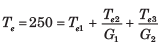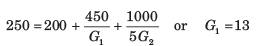Test: Noise - Question 4

An amplifier has an operating spot noise figure of 10 dB when driven by a source of effective noise temperature 225 K.

Que: The standard spot noise figure of amplifier is

Detailed Solution for Test: Noise - Question 4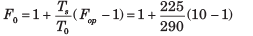= 7.98 or 9.0 dB

Test: Noise - Question 5

An amplifier has an operating spot noise figure of 10 dB when driven by a source of effective noise temperature 225 K.

Que: If a matched attenuator with a loss of 3.2 dB isplaced between the source and the amplifier's input,what is the operating spot noise figure of the attenuator's amplifier cascade if the attenuator's physicaltemperature is 290 K ?

Detailed Solution for Test: Noise - Question 5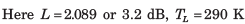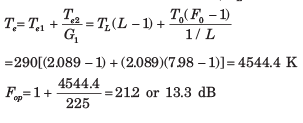Test: Noise - Question 6

An amplifier has an operating spot noise figure of 10 dB when driven by a source of effective noise temperature 225 K.

Que: In previous question what is the standard spot noisefigure of the cascade ?

Detailed Solution for Test: Noise - Question 6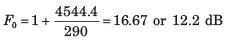Test: Noise - Question 7

Omega Electronics sells a microwave receiver

(A) having an operating spot noise figure of 10 dB when driven by a source with effective noise temperature 130 K Digilink

(B) sells a receiver with a standard spot noise figure of 6 dB. Microtronics

(C) sells a receiver with standard spot noise figure of 8 dB when driven by a source with effective noise temperature 190 K. The best receiver to purchase is

Detailed Solution for Test: Noise - Question 7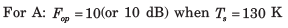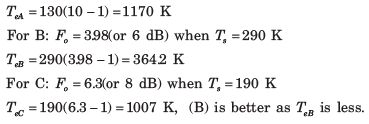Test: Noise - Question 8

An amplifier has three stages for which Te1 = 150 K (first stage), Te3 = 350 K, and Te3 = 600 K (output stage). Available power gain of the first stage is 10 and overall input effective noise temperature is 190 K

Que: The available power gain of the second stage is

Detailed Solution for Test: Noise - Question 8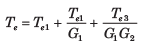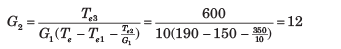Test: Noise - Question 9

An amplifier has three stages for which Te1 = 150 K (first stage), Te3 = 350 K, and Te3 = 600 K (output stage). Available power gain of the first stage is 10 and overall input effective noise temperature is 190 K

Que: The cascade's standard spot noise figure is

Detailed Solution for Test: Noise - Question 9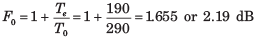Test: Noise - Question 10

An amplifier has three stages for which Te1 = 150 K (first stage), Te3 = 350 K, and Te3 = 600 K (output stage). Available power gain of the first stage is 10 and overall input effective noise temperature is 190 K

Que: What is the cascade's operating spot noise figure when used with a source of noise temperature Ts = 50 K

Detailed Solution for Test: Noise - Question 10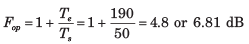Test: Noise - Question 11

Three network are cascaded. Available power gains are G1 = 8, G2 = 6 and G3 = 20. Respective input effective spot noise temperature are Te1 = 40 K, Te2 = 100 K and Te3 =180 K.

Detailed Solution for Test: Noise - Question 11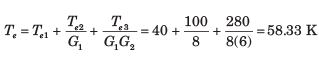Test: Noise - Question 12

Three identical amplifier, each having a spot effective input noise temperature of 125 K and available power G are cascaded. The overall spot effective input noise temperature of the cascade is 155 K. The G is

Detailed Solution for Test: Noise - Question 12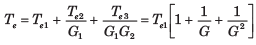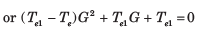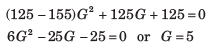Test: Noise - Question 13

Three amplifier that may be connected in any order in a cascade are defined as follows: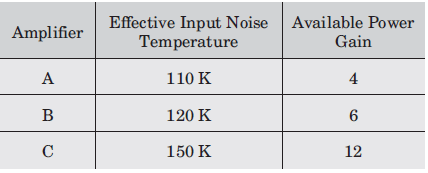The sequence of connection that will give the lowest overall effective input noise temperature for the cascade is

Detailed Solution for Test: Noise - Question 13

Sequence Te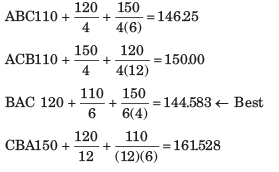Test: Noise - Question 14

What is the maximum average effective input noise temperature that an amplifier can have if its average standard noise figure is to not exceed 1.7 ?

Detailed Solution for Test: Noise - Question 14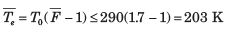Test: Noise - Question 15

An amplifier has an average standard noise figure of 2.0 dB and an average operating noise figure of 6.5 dB when used with a source of average effective source temperature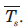Theis

Detailed Solution for Test: Noise - Question 15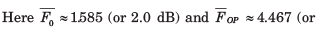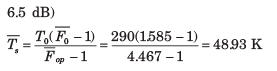Test: Noise - Question 16

An antenna with average noise temperature 60 K connects to a receiver through various microwave elements that can be modeled as an impedance matched attenuator with an overall loss of 2.4 dB and a physical temperatures of 275 K. The overall system noise temperature of the receiver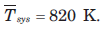Que: The average effective input noise temperature of the receiver is

Detailed Solution for Test: Noise - Question 16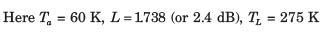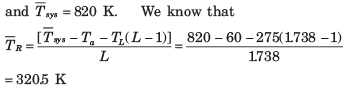Test: Noise - Question 17

An antenna with average noise temperature 60 K connects to a receiver through various microwave elements that can be modeled as an impedance matched attenuator with an overall loss of 2.4 dB and a physical temperatures of 275 K. The overall system noise temperature of the receiverDetailed Solution for Test: Noise - Question 17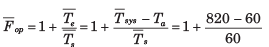= 13.67 or 11.4 dB

Test: Noise - Question 18

If receiver has an available power gain of 110 dB and a noise band width of 10 MHz, the available output noise power of receiver is

Detailed Solution for Test: Noise - Question 18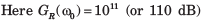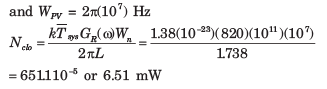Test: Noise - Question 19

If antenna attenuator cascade is considered as a noise source, its average effective noise temperature is

Detailed Solution for Test: Noise - Question 19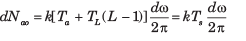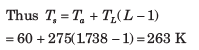Test: Noise - Question 20

An amplifier when used with a source of average oise temperature 60 K, has an average operating noise figure of 5.
Que: The Te is

Detailed Solution for Test: Noise - Question 20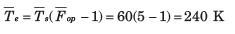## GATE ECE (Electronics) 2024 Mock Test Series

22 docs|263 tests